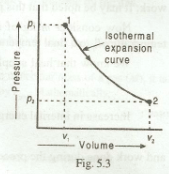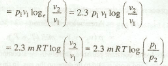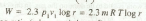# Constant Temperature | Isothermal Process

A process, in which the temperature of the working substance remains constant during its expansion or compression, is called a constant temperature process or isothermal process. This will happen when the working substance remains in a perfect thermal contact with the surroundings, so that the heat 'sucked in' or 'squeezed out' is compensated exactly for the mechanical work done by, or on the gas respectively. It is thus obvious that in an isothermal process:

1. there is no change in temperature, and
2. there is no change in internal energy.

We know that

Q1-2 = dU + W1-2 = W1-2

Hence during isothermal expansion of a gas,

Heat added = Work done by the gas

Similarly, during isothermal compression of a gas,

Heat subtracted = Work done on the gas

A little consideration will show that the isothermal process is governed by Boyle's law. Thus the isothermal equation of a perfect gas is pv = Constant.

Now consider a certain quantity of a perfect gas being expanded isothermally, which is shown by the curve 1-2 in Fig. 5.3.Let
v1 = Initial volume of gas,
p1 = Initial pressure of gas,
v2 = Final volume of gas, and
p2 = Final pressure of gas.

The workdone during isothermal expansion is given by

W1-2 = Area under the curve 1-2In general,where r is the expansion ratio (when gas is heated) or compression ratio (when gas is cooled).

Notes:

(a) Expansion ratio (r) = (Volume at the end of expansion)/(Volume at the beginning of expansion)
(b) Compression ratio (r) = (Volume at the beginning of compression)/(Volume at the end of compression)